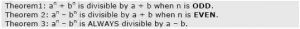HomeCAT & Non-CATCAT ExamPast CAT Remainder Questions

# Past CAT Remainder Questions

So we have this biggest community for CAT Preparation, and we discuss almost everything about CAT here in this group called CAT Preparation – iQuanta .Anyone can be a part of it by sending a join request.

So here are few variety of questions on Past CAT Remainder Questions, discussed in group along with detailed solutions.

1.What is the remainder when 4^96 is divided by 6?

1) 0
2) 2
3) 3
4) 4

Remark: It can be done in 3 different ways, iQuanta shortcut, or Euler or Chinese remainder Theorem

2. The remainder, when (15^23 + 23^23) is divided by 19, is:

1) 4
2) 15
3) 0
4) 18

Remark : It uses3. If x = (16^3 + 17^3 + 18^3 + 19^3), then x divided by 70 leaves a remainder of

1) 0
2) 1
3) 69
4) 35

Remark : It uses concept of previous questions, but it has 1 extra condition to remember.

4. Let n! = 1 × 2 × 3 × … × n for integer n ≥ 1. If p = 1! + (2 × 2!) + (3 × 3!) + … +(10 × 10!), then p + 2 when divided by 11! leaves a remainder of

1) 10
2) 0
3) 7
4) 1

Remark : It has 2 ways : One by general expanding and other is a generalised shortcut, both discussed in the link below

5. Let N = 1421 × 1423 × 1425. What is the remainder when N is divided by 12?

1) 0
2) 9
3) 3
4) 6

Remark : It is one of the easiest questions on remainder asked in CAT.

6. The integers 34041 and 32506 when divided by a three-digit integer ‘n’ leave the same remainder. What is ‘n’?

Remark : It is a very conceptual question and tricky one if one doesn’t know the solution already.

7. On dividing a number by 3, 4 and 7, the remainders are 2, 1 and 4 respectively. If the same number is divided by 84 then the remainder is

1) 80
2) 76
3) 53
4) None of these

Remark : A question on Chinese remainder theorem

8. For all integers n > 0, 7^6n – 6^6n is divisible by

1) 13
2) 127
3) 559
4) All of these

Remark: An application of algebraic formula a^2-b^2=(a-b)(a+b)

9. The remainder when 2^256 is divided by 17 is

1) 7
2) 13
3) 11
4) 1

Remark : A simple question on Euler theorem

10. 101! Mod 103 [ CAT 2003]

Remark : A simple question on Wilson Theorem

## More from Author

### 7 Reasons Why Mock Tests Are Important For CAT 2023 Preparation

Every CAT aspirant has heard about mock tests. Yet they are...

### 10 Tips To Stay Self-Motivated During Your Exam Preparation

If I gave you easy to follow 10 tips to stay...

### Top 10 Reasons You Should Start Preparing For CAT Exam 2023 Early

If you are a CAT aspirant and confused about when to...